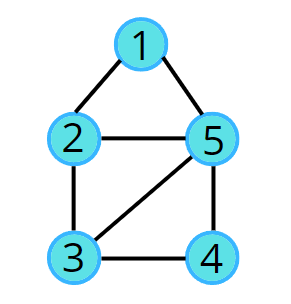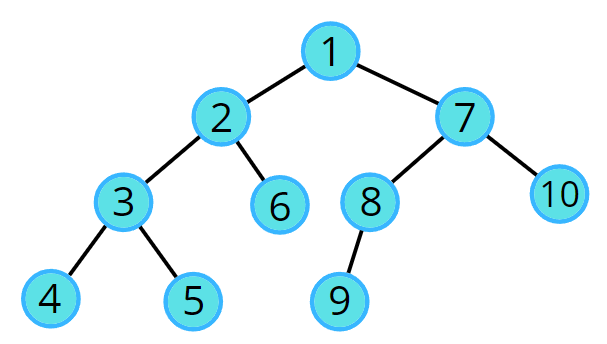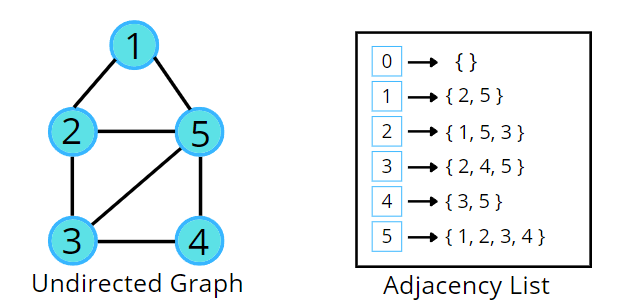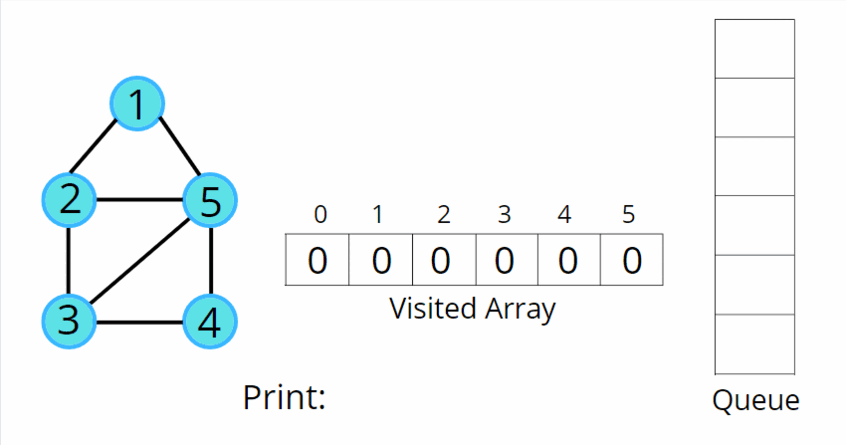# Breadth First Search (BFS): Level Order Traversal

Problem Statement: Given an undirected graph, return a vector of all nodes by traversing the graph using breadth-first search (BFS).

Pre-req: Graph Representation, Queue STL

Examples:

```Example 1:
Input:Output: 1 2 5 3 4```
```Example 2:
Input:Output: 1 2 7 3 6 8 10 4 5 9```

### Solution

Disclaimer: Don’t jump directly to the solution, try it out yourself first.

### Approach:

Initial Configuration:

• Queue data structure: follows FIFO, and will always contain the starting.
• Visited array: an array initialized to 0
1. In BFS, we start with a “starting” node, mark it as visited, and push it into the queue data structure.
2. In every iteration, we pop out the node ‘v’ and put it in the solution vector, as we are traversing this node.
3. All the unvisited adjacent nodes from ‘v’ are visited next and are pushed into the queue. The list of adjacent neighbors of the node can be accessed from the adjacency list.
4. Repeat steps 2 and 3 until the queue becomes empty, and this way you can easily traverse all the nodes in the graph.

In this way, all the nodes are traversed in a breadthwise manner.Code:

## C++ Code

``````#include <bits/stdc++.h>
using namespace std;

class Solution {
public:
// Function to return Breadth First Traversal of given graph.
vector<int> bfsOfGraph(int V, vector<int> adj[]) {
int vis[V] = {0};
vis = 1;
queue<int> q;
// push the initial starting node
q.push(0);
vector<int> bfs;
// iterate till the queue is empty
while(!q.empty()) {
// get the topmost element in the queue
int node = q.front();
q.pop();
bfs.push_back(node);
// traverse for all its neighbours
for(auto it : adj[node]) {
// if the neighbour has previously not been visited,
// store in Q and mark as visited
if(!vis[it]) {
vis[it] = 1;
q.push(it);
}
}
}
return bfs;
}
};

void addEdge(vector <int> adj[], int u, int v) {
}

void printAns(vector <int> &ans) {
for (int i = 0; i < ans.size(); i++) {
cout << ans[i] << " ";
}
}

int main()
{

Solution obj;
vector <int> ans = obj.bfsOfGraph(5, adj);
printAns(ans);

return 0;
}
``````

Output: 0 1 4 2 3

Time Complexity: O(N) + O(2E), Where N = Nodes, 2E is for total degrees as we traverse all adjacent nodes.

Space Complexity: O(3N) ~ O(N), Space for queue data structure visited array and an adjacency list

## Java Code

``````import java.util.*;
class Solution {
// Function to return Breadth First Traversal of given graph.
public ArrayList<Integer> bfsOfGraph(int V,

ArrayList < Integer > bfs = new ArrayList < > ();
boolean vis[] = new boolean[V];
Queue < Integer > q = new LinkedList < > ();

vis = true;

while (!q.isEmpty()) {
Integer node = q.poll();

// Get all adjacent vertices of the dequeued vertex s
// If a adjacent has not been visited, then mark it
// visited and enqueue it
for (Integer it: adj.get(node)) {
if (vis[it] == false) {
vis[it] = true;
}
}
}

return bfs;
}

public static void main(String args[]) {

ArrayList < ArrayList < Integer >> adj = new ArrayList < > ();
for (int i = 0; i < 5; i++) {
}

Solution sl = new Solution();
ArrayList < Integer > ans = sl.bfsOfGraph(5, adj);
int n = ans.size();
for(int i = 0;i<n;i++) {
System.out.print(ans.get(i)+" ");
}
}
}
``````

Output: 0 1 4 2 3

Time Complexity: O(N) + O(2E), Where N = Nodes, 2E is for total degrees as we traverse all adjacent nodes.

Space Complexity: O(3N) ~ O(N), Space for queue data structure visited array and an adjacency list

Special thanks to Vanshika Singh Gour for contributing to this article on takeUforward. If you also wish to share your knowledge with the takeUforward fam, please check out this articleIf you want to suggest any improvement/correction in this article please mail us at [email protected]